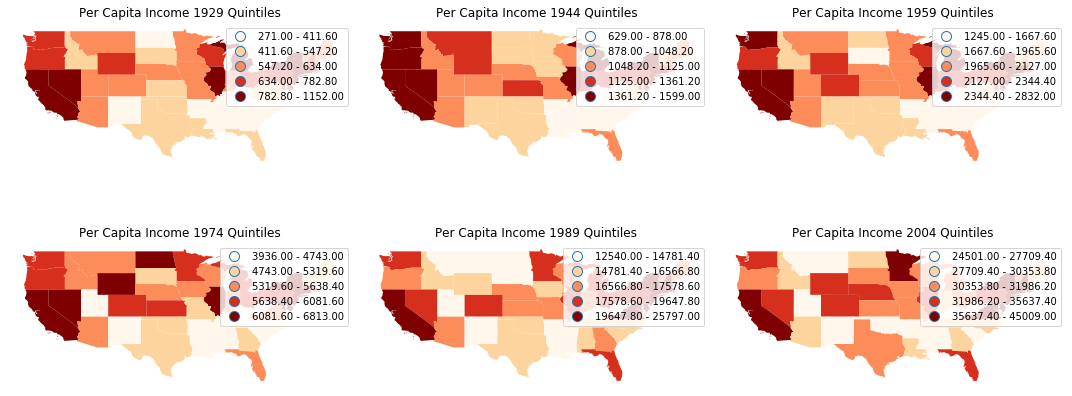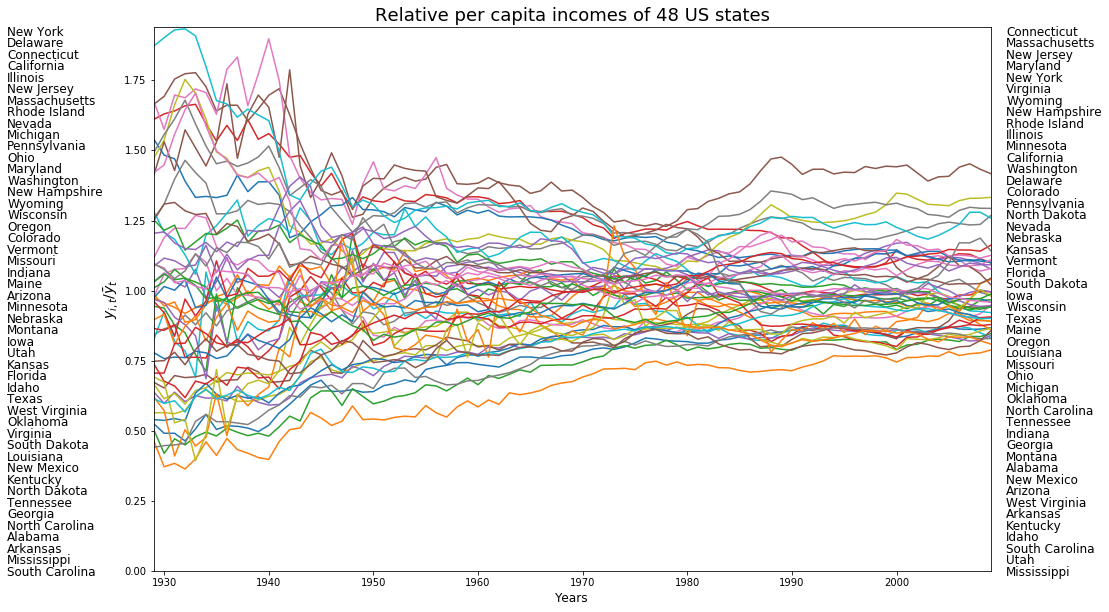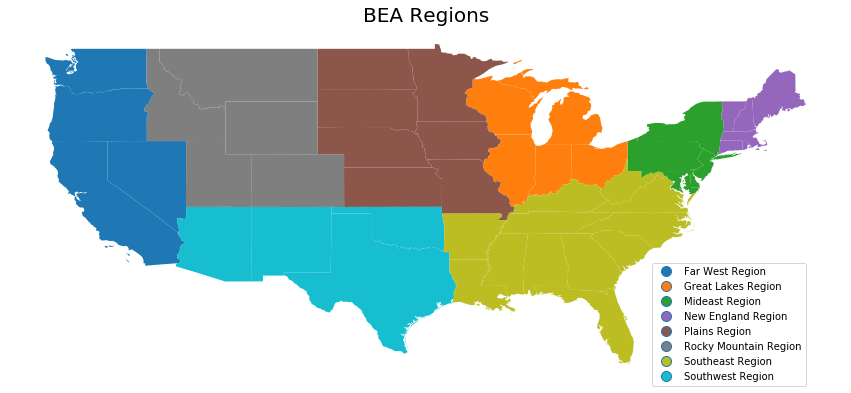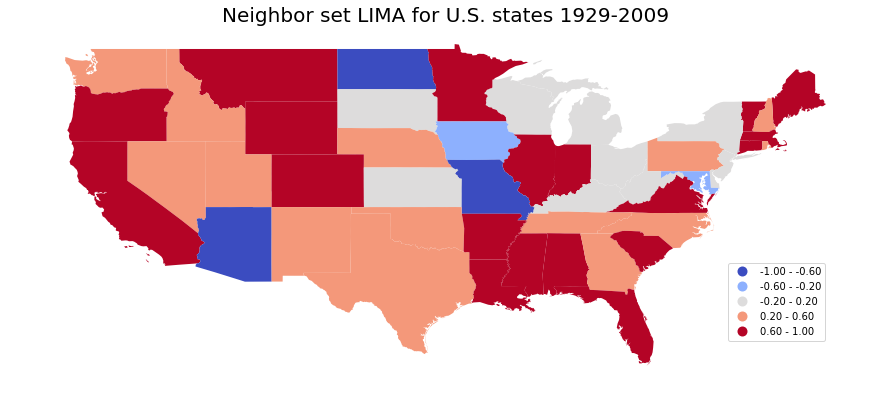# Rank based Methods¶

Author: Wei Kang [email protected], Serge Rey [email protected]

## Introduction¶

This notebook introduces two classic nonparametric statistics of exchange mobility and their spatial extensions. We will demonstrate the usage of these methods by an empirical study for understanding regional exchange mobility pattern in US. The dataset is the per capita incomes observed annually from 1929 to 2010 for the lower 48 US states.

## Regional exchange mobility pattern in US 1929-2009¶

Firstly we load in the US dataset:

In :
import pandas as pd
import libpysal
import geopandas as gpd
import numpy as np

complete_table = geo_table.merge(income_table,left_on='STATE_NAME',right_on='Name')

Out:
AREA PERIMETER STATE_ STATE_ID STATE_NAME STATE_FIPS_x SUB_REGION STATE_ABBR geometry Name ... 2000 2001 2002 2003 2004 2005 2006 2007 2008 2009
0 20.750 34.956 1 1 Washington 53 Pacific WA (POLYGON ((-122.400749206543 48.22539520263672... Washington ... 31528 32053 32206 32934 34984 35738 38477 40782 41588 40619
1 45.132 34.527 2 2 Montana 30 Mtn MT POLYGON ((-111.4746322631836 44.70223999023438... Montana ... 22569 24342 24699 25963 27517 28987 30942 32625 33293 32699
2 9.571 18.899 3 3 Maine 23 N Eng ME (POLYGON ((-69.77778625488281 44.0740737915039... Maine ... 25623 27068 27731 28727 30201 30721 32340 33620 34906 35268
3 21.874 21.353 4 4 North Dakota 38 W N Cen ND POLYGON ((-98.73005676269531 45.93829727172852... North Dakota ... 25068 26118 26770 29109 29676 31644 32856 35882 39009 38672
4 22.598 22.746 5 5 South Dakota 46 W N Cen SD POLYGON ((-102.7879333496094 42.99532318115234... South Dakota ... 26115 27531 27727 30072 31765 32726 33320 35998 38188 36499

5 rows × 92 columns

We will visualize the spatial distributions of per capita incomes of US states across 1929 to 2009 to obtain a first impression of the dynamics.

In :
import matplotlib.pyplot as plt
%matplotlib inline

index_year = range(1929,2010,15)
fig, axes = plt.subplots(nrows=2, ncols=3,figsize = (15,7))
for i in range(2):
for j in range(3):
ax = axes[i,j]
complete_table.plot(ax=ax, column=str(index_year[i*3+j]), cmap='OrRd', scheme='quantiles', legend=True)
ax.set_title('Per Capita Income %s Quintiles'%str(index_year[i*3+j]))
ax.axis('off')
plt.tight_layout()In :
years = range(1929,2010)
names = income_table['Name']
pci = income_table.drop(['Name','STATE_FIPS'], 1).values.T
rpci= (pci.T / pci.mean(axis=1)).T
order1929 = np.argsort(rpci[0,:])
order2009 = np.argsort(rpci[-1,:])
names1929 = names[order1929[::-1]]
names2009 = names[order2009[::-1]]
first_last = np.vstack((names1929,names2009))
from pylab import rcParams
rcParams['figure.figsize'] = 15,10
p = plt.plot(years,rpci)
for i in range(48):
plt.text(1915,1.91-(i*0.041), first_last[i],fontsize=12)
plt.text(2010.5,1.91-(i*0.041), first_last[i],fontsize=12)
plt.xlim((years, years[-1]))
plt.ylim((0, 1.94))
plt.ylabel(r"$y_{i,t}/\bar{y}_t$",fontsize=14)
plt.xlabel('Years',fontsize=12)
plt.title('Relative per capita incomes of 48 US states',fontsize=18)

Out:
Text(0.5, 1.0, 'Relative per capita incomes of 48 US states')The above figure displays the trajectories of relative per capita incomes of 48 US states. It is quite obvious that states were swapping positions across 1929-2009. We will demonstrate how to quantify the exchange mobility as well as how to assess the regional and local contribution to the overall exchange mobility. We will ultilize BEA regions and base on it for constructing the block weight matrix.

BEA regional scheme divide US states into 8 regions:

• New England Region
• Mideast Region
• Great Lakes Region
• Plains Region
• Southeast Region
• Southwest Region
• Rocky Mountain Region
• Far West Region

As the dataset does not contain information regarding BEA regions, we manually input the regional information:

In :
BEA_regions = ["New England Region","Mideast Region","Great Lakes Region","Plains Region","Southeast Region","Southwest Region","Rocky Mountain Region","Far West Region"]
BEA_regions_abbr = ["NENG","MEST","GLAK","PLNS","SEST","SWST","RKMT","FWST"]
BEA = pd.DataFrame({ 'Region code' : np.arange(1,9,1), 'BEA region' : BEA_regions,'BEA abbr':BEA_regions_abbr})
BEA

Out:
Region code BEA region BEA abbr
0 1 New England Region NENG
1 2 Mideast Region MEST
2 3 Great Lakes Region GLAK
3 4 Plains Region PLNS
4 5 Southeast Region SEST
5 6 Southwest Region SWST
6 7 Rocky Mountain Region RKMT
7 8 Far West Region FWST
In :
region_code = list(np.repeat(1,6))+list(np.repeat(2,6))+list(np.repeat(3,5))+list(np.repeat(4,7))+list(np.repeat(5,12))+list(np.repeat(6,4))+list(np.repeat(7,5))+list(np.repeat(8,6))
state_code = ['09','23','25','33','44','50','10','11','24','34','36','42','17','18','26','39','55','19','20','27','29','31','38','46','01','05','12','13','21','22','28','37','45','47','51','54','04','35','40','48','08','16','30','49','56','02','06','15','32','41','53']
state_region = pd.DataFrame({'Region code':region_code,"State code":state_code})
state_region_all = state_region.merge(BEA,left_on='Region code',right_on='Region code')
complete_table = complete_table.merge(state_region_all,left_on='STATE_FIPS_x',right_on='State code')

Out:
AREA PERIMETER STATE_ STATE_ID STATE_NAME STATE_FIPS_x SUB_REGION STATE_ABBR geometry Name ... 2004 2005 2006 2007 2008 2009 Region code State code BEA region BEA abbr
0 20.750 34.956 1 1 Washington 53 Pacific WA (POLYGON ((-122.400749206543 48.22539520263672... Washington ... 34984 35738 38477 40782 41588 40619 8 53 Far West Region FWST
1 45.132 34.527 2 2 Montana 30 Mtn MT POLYGON ((-111.4746322631836 44.70223999023438... Montana ... 27517 28987 30942 32625 33293 32699 7 30 Rocky Mountain Region RKMT
2 9.571 18.899 3 3 Maine 23 N Eng ME (POLYGON ((-69.77778625488281 44.0740737915039... Maine ... 30201 30721 32340 33620 34906 35268 1 23 New England Region NENG
3 21.874 21.353 4 4 North Dakota 38 W N Cen ND POLYGON ((-98.73005676269531 45.93829727172852... North Dakota ... 29676 31644 32856 35882 39009 38672 4 38 Plains Region PLNS
4 22.598 22.746 5 5 South Dakota 46 W N Cen SD POLYGON ((-102.7879333496094 42.99532318115234... South Dakota ... 31765 32726 33320 35998 38188 36499 4 46 Plains Region PLNS

5 rows × 96 columns

The BEA regions are visualized below:

In :
fig, ax = plt.subplots(nrows=1, ncols=1,figsize = (15,8))
beaplot = complete_table.plot(ax=ax,column="BEA region", legend=True)
leg = ax.get_legend()
leg.set_bbox_to_anchor((0.8, 0.15, 0.16, 0.2))
beaplot.set_title("BEA Regions",fontdict={"fontsize":20})
ax.set_axis_off()## Kendall's Tau¶

Kendall’s $\tau$ statistic is based on a comparison of the number of pairs of $n$ observations that have concordant ranks between two variables. For measuring exchange mobility in giddy, the two variables in question are the values of an attribute measured at two points in time over $n$ spatial units. This classic measure of rank correlation indicates how much relative stability there has been in the map pattern over the two periods. Spatial decomposition of Kendall’s $\tau$ could be classified into three spatial scales: global spatial decomposition , inter- and intra-regional decomposition and local spatial decomposition. More details will be given latter.

### Classic Kendall Tau¶

Kendall's $\tau$ statistic is a global measure of exchange mobility. For $n$ spatial units over two periods, it is formally defined as follows:

\begin{equation} \tau = \frac{c-d}{(n(n-1))/2} \end{equation}

where $c$ is the number of concordant pairs (two spatial units which do not exchange ranks over two periods), and $d$ is the number of discordant pairs (two spatial units which exchange ranks over two periods). $-1 \leq \tau \leq 1$. Smaller $\tau$ indicates higher exchange mobility.

In giddy, class $Tau$ requires two inputs: a cross-section of income values at one period ($x$) and a cross-section of income values at another period ($y$):

giddy.rank.Tau(self, x, y)


We will construct a $Tau$ instance by specifying the incomes in two periods. Here, we look at the global exchange mobility of US states between 1929 and 2009.

In :
import giddy

In :
tau = giddy.rank.Tau(complete_table["1929"],complete_table["2009"])
tau

Out:
<giddy.rank.Tau at 0x1a2b64e210>
In :
tau.concordant

Out:
856.0
In :
tau.discordant

Out:
271.0

There are 856 concordant pairs of US states between 1929 and 2009, and 271 discordant pairs.

In :
tau.tau

Out:
0.5188470576690462
In :
tau.tau_p

Out:
1.9735720263920198e-07

The observed Kendall's $\tau$ statistic is 0.519 and its p-value is $1.974 \times 10^{-7}$. Therefore, we will reject the null hypothesis of no assocation between 1929 and 2009 at the $5\%$ significance level.

### Spatial Kendall Tau¶

The spatial Kendall's $\tau$ decomposes all pairs into those that are spatial neighbors and those that are not, and examines whether the rank correlation is different between the two sets (Rey, 2014).

\begin{equation} \tau_w = \frac{\iota'(W\circ S)\iota}{\iota'W \iota} \end{equation}

$W$ is the spatial weight matrix, $S$ is the concordance matrix and $\iota$ is the $(n,1)$ unity vector. The null hypothesis is the spatial randomness of rank exchanges. The inference of $\tau_w$ could be conducted based on random spatial permutation of incomes at two periods.

giddy.rank.SpatialTau(self, x, y, w, permutations=0)


For illustration, we turn back to the case of incomes in US states over 1929-2009:

In :
from libpysal.weights import block_weights
w = block_weights(complete_table["BEA region"])
np.random.seed(12345)
tau_w = giddy.rank.SpatialTau(complete_table["1929"],complete_table["2009"],w,999)

/Users/weikang/Google Drive/python_repos/pysal-refactor/libpysal/libpysal/weights/weights.py:165: UserWarning: The weights matrix is not fully connected:
There are 8 disconnected components.
warnings.warn(message)

In :
tau_w.concordant

Out:
856.0
In :
tau_w.concordant_spatial

Out:
103
In :
tau_w.discordant

Out:
271.0
In :
tau_w.discordant_spatial

Out:
41

Out of 856 concordant pairs of spatial units, 103 belong to the same region (and are considered neighbors); out of 271 discordant pairs of spatial units, 41 belong to the same region.

In :
tau_w.tau_spatial

Out:
0.4305555555555556
In :
tau_w.tau_spatial_psim

Out:
0.001

The estimate of spatial Kendall's $\tau$ is 0.431 and its p-value is 0.001 which is much smaller than the significance level $0.05$. Therefore, we reject the null of spatial randomness of exchange mobility. The fact that $\tau_w=0.431$ is smaller than the global average $\tau=0.519$ implies that globally a significant number of rank exchanges happened between states within the same region though we do not know the specific region or regions hosting these rank exchanges. A more thorough decomposition of $\tau$ such as inter- and intra-regional indicators and local indicators will provide insights on this issue.

### Inter- and Intra-regional decomposition of Kendall's Tau¶

A meso-level view on the exchange mobility pattern is provided by inter- and intra-regional decomposition of Kendall's $\tau$. This decomposition can shed light on specific regions hosting most rank exchanges. More precisely, insteading of examining the concordance relationship between any two neighboring spatial units in the whole study area, for a specific region A, we examine the concordance relationship between any two spatial units within region A (neighbors), resulting in the intraregional concordance statistic for A; or we could examine the concordance relationship between any spatial unit in region A and any spatial unit in region B (nonneighbors), resulting in the interregional concordance statistic for A and B. If there are k regions, there will be k intraregional concordance statistics and $(k-1)^2$ interregional concordance statistics, we could organize them into a $(k,k)$ matrix where the diagonal elements are intraregional concordance statistics and nondiagnoal elements are interregional concordance statistics.

Formally, this inter- and intra-regional concordance statistic matrix is defined as follows (Rey, 2016):

\begin{equation} T=\frac{P(H \circ S)P'}{P H P'} \end{equation}

$P$ is a $(k,n)$ binary matrix where $p_{j,i}=1$ if spatial unit $i$ is in region $j$ and $p_{j,i}=0$ otherwise. $H$ is a $(n,n)$ matrix with 0 on diagnoal and 1 on other places. $\circ$ is the Hadamard product. Inference could be based on random spatial permutation of incomes at two periods, similar to spatial $\tau$.

To obtain an estimate for the inter- and intra-regional indicator matrix, we use the $Tau\_Regional$ class:

giddy.rank.Tau_Regional(self, x, y, regime, permutations=0)


Here, $regime$ is an 1-dimensional array of size n. Each element is the id of which region an spatial unit belongs to.

In :
giddy.rank.Tau_Regional?


Similar to before, we go back to the case of incomes in US states over 1929-2009:

In :
np.random.seed(12345)
tau_w = giddy.rank.Tau_Regional(complete_table["1929"],complete_table["2009"],complete_table["BEA region"],999)
tau_w

/Users/weikang/Google Drive (weikang@ucr.edu)/python_repos/pysal-refactor/libpysal/libpysal/weights/weights.py:170: UserWarning: The weights matrix is not fully connected. There are 8 components
warnings.warn("The weights matrix is not fully connected. There are %d components" % self.n_components)

Out:
<giddy.rank.Tau_Regional at 0x1a212d85c0>
In :
tau_w.tau_reg

Out:
array([[ 0.66666667,  0.5       ,  0.3       ,  0.41666667,  0.28571429,
0.5       ,  0.79166667,  0.875     ],
[ 0.5       ,  0.4       ,  0.52      ,  0.26666667, -0.48571429,
0.52      ,  0.53333333,  0.6       ],
[ 0.3       ,  0.52      ,  0.        ,  0.4       ,  0.88571429,
0.76      ,  0.93333333,  1.        ],
[ 0.41666667,  0.26666667,  0.4       ,  0.86666667,  0.47619048,
0.83333333,  0.86111111,  0.91666667],
[ 0.28571429, -0.48571429,  0.88571429,  0.47619048, -0.14285714,
0.42857143,  0.69047619,  0.14285714],
[ 0.5       ,  0.52      ,  0.76      ,  0.83333333,  0.42857143,
0.8       ,  0.06666667,  0.1       ],
[ 0.79166667,  0.53333333,  0.93333333,  0.86111111,  0.69047619,
0.06666667,  0.54545455,  0.33333333],
[ 0.875     ,  0.6       ,  1.        ,  0.91666667,  0.14285714,
0.1       ,  0.33333333,  0.        ]])

The attribute $tau\_reg$ gives the inter- and intra-regional concordance statistic matrix. Higher values represents lower exchange mobility. Obviously there are some negative values indicating high exchange mobility. Attribute $tau\_reg\_pvalues$ gives pvalues for all inter- and intra-regional concordance statistics:

In :
tau_w.tau_reg_pvalues

Out:
array([[0.586, 0.516, 0.196, 0.37 , 0.151, 0.526, 0.051, 0.104],
[0.516, 0.41 , 0.583, 0.114, 0.001, 0.532, 0.526, 0.472],
[0.196, 0.583, 0.102, 0.316, 0.011, 0.156, 0.001, 0.014],
[0.37 , 0.114, 0.316, 0.122, 0.41 , 0.034, 0.003, 0.026],
[0.151, 0.001, 0.011, 0.41 , 0.013, 0.344, 0.08 , 0.051],
[0.526, 0.532, 0.156, 0.034, 0.344, 0.324, 0.005, 0.056],
[0.051, 0.526, 0.001, 0.003, 0.08 , 0.005, 0.502, 0.136],
[0.104, 0.472, 0.014, 0.026, 0.051, 0.056, 0.136, 0.166]])

We can manipulate these two attribute to obtain significant inter- and intra-regional statistics only (at the $5\%$ significance level):

In :
tau_w.tau_reg * (tau_w.tau_reg_pvalues<0.05)

Out:
array([[ 0.        ,  0.        ,  0.        ,  0.        ,  0.        ,
0.        ,  0.        ,  0.        ],
[ 0.        ,  0.        ,  0.        ,  0.        , -0.48571429,
0.        ,  0.        ,  0.        ],
[ 0.        ,  0.        ,  0.        ,  0.        ,  0.88571429,
0.        ,  0.93333333,  1.        ],
[ 0.        ,  0.        ,  0.        ,  0.        ,  0.        ,
0.83333333,  0.86111111,  0.91666667],
[ 0.        , -0.48571429,  0.88571429,  0.        , -0.14285714,
0.        ,  0.        ,  0.        ],
[ 0.        ,  0.        ,  0.        ,  0.83333333,  0.        ,
0.        ,  0.06666667,  0.        ],
[ 0.        ,  0.        ,  0.93333333,  0.86111111,  0.        ,
0.06666667,  0.        ,  0.        ],
[ 0.        ,  0.        ,  1.        ,  0.91666667,  0.        ,
0.        ,  0.        ,  0.        ]])

The table below displays the inter- and intra-regional decomposition matrix of Kendall's $\tau$ for US states over 1929-2009 based on the 8 BEA regions. Bold numbers indicate significance at the $5\%$ significance level. The negative and significant intra-Southeast concordance statistic ($-0.486$) indicates that the rank exchanges within Southeast region is significantly more frequent than those between states within and out of Southeast region.

Region New England Mideast Great Lakes Plains Southeast Southwest Rocky Mountain Far West
New England 0.667 0.5 0.3 0.417 0.2856 0.5 0.792 0.875
Mideast 0.5 0.4 0.52 0.267 -0.486 0.52 0.533 0.6
Great Lakes 0.3 0.52 0 0.4 0.886 0.76 0.933 1.
Plains 0.417 0.267 0.4 0.867 0.476 0.833 0.861 0.917
Southeast 0.286 -0.486 0.886 0.476 -0.143 0.429 0.690 0.143
Southwest 0.5 0.52 0.76 0.833 0.429 0.8 0.067 0.1
Rocky Mountain 0.792 0.533 0.933 0.861 0.69 0.067 0.545 0.333
Far West 0.875 0.6 1. 0.917 0.143 0.1 0.333 0

### Local Kendall Tau¶

Local Kendall's $\tau$ is a local decomposition of classic Kendall's $\tau$ which provides an indication of the contribution of spatial unit $r$’s rank changes to the overall level of exchange mobility (Rey, 2016). Focusing on spatial unit $r$, we formally define it as follows:

\begin{equation} \tau_{r} = \frac{c_r - d_r}{n-1} \end{equation}

where $c_r$ is the number of spatial units (except $r$) which are concordant with $r$ and $d_r$ is the number of spatial units which are discordant with $r$. Similar to classic Kendall's $\tau$, local $\tau$ takes values on $[-1,1]$. The larger the value, the lower the exchange mobility for $r$.

giddy.rank.Tau_Local(self, x, y)


We create a $Tau\_Local$ instance for US dynamics 1929-2009:

In :
tau_r = giddy.rank.Tau_Local(complete_table["1929"],complete_table["2009"])
tau_r

Out:
<giddy.rank.Tau_Local at 0x1141187b8>
In :
pd.DataFrame({"STATE_NAME":complete_table['STATE_NAME'].tolist(),"$\\tau_r$":tau_r.tau_local}).head()

Out:
STATE_NAME $\tau_r$
0 Washington 0.617021
1 Montana 0.446809
2 Maine 0.404255
3 North Dakota -0.021277
4 South Dakota 0.319149

Therefore, local concordance measure produces a negative value for North Dakota (-0.0213) indicating that North Dakota exchanged ranks with a lot of states across 1929-2000. On the contrary, the local $\tau$ statistic is quite high for Washington (0.617) highlighting a high stability of Washington.

### Local indicator of mobility association-LIMA¶

To reveal the role of space in shaping the exchange mobility pattern for each spatial unit, two spatial variants of local Kendall's $\tau$ could be utilized: neighbor set LIMA and neighborhood set LIMA (Rey, 2016). The latter is also the result of a decomposition of local Kendall's $\tau$ (into neighboring and nonneighboring parts) as well as a decompostion of spatial Kendall's $\tau$ (into its local components).

#### Neighbor set LIMA¶

Instead of examining the concordance relationship between a focal spatial unit $r$ and all the other units as what local $\tau$ does, neighbor set LIMA focuses on the concordance relationship between a focal spatial unit $r$ and its neighbors only. It is formally defined as follows:

\begin{equation} \tilde{\tau}_{r} = \frac{\sum_b w_{r,b} s_{r,b}}{\sum_b w_{r,b}} \end{equation}
giddy.rank.Tau_Local_Neighbor(self, x, y, w, permutations=0)

In :
tau_wr = giddy.rank.Tau_Local_Neighbor(complete_table["1929"],complete_table["2009"],w,999)
tau_wr

Out:
<giddy.rank.Tau_Local_Neighbor at 0x114118f98>
In :
tau_wr.tau_ln

Out:
array([ 0.33333333,  1.        ,  1.        , -0.66666667,  0.        ,
1.        ,  0.        ,  0.5       ,  1.        ,  0.66666667,
1.        ,  0.6       , -0.33333333,  1.        ,  0.33333333,
0.        ,  0.5       ,  1.        ,  0.6       ,  0.        ,
1.        ,  0.33333333,  0.5       ,  1.        ,  0.        ,
1.        ,  0.        , -0.09090909, -0.5       ,  1.        ,
0.09090909,  0.        ,  0.63636364, -1.        , -1.        ,
0.33333333,  0.27272727,  0.45454545,  0.33333333,  0.33333333,
0.63636364,  0.81818182,  0.45454545,  0.81818182,  0.81818182,
0.81818182,  0.81818182,  0.        ])

To visualize the spatial distribution of neighbor set LIMA:

In :
complete_table["tau_ln"] =tau_wr.tau_ln
fig, ax = plt.subplots(nrows=1, ncols=1,figsize = (15,8))
ln_map = complete_table.plot(ax=ax, column="tau_ln", cmap='coolwarm', scheme='equal_interval',legend=True)
leg = ax.get_legend()
leg.set_bbox_to_anchor((0.8, 0.15, 0.16, 0.2))
ln_map.set_title("Neighbor set LIMA for U.S. states 1929-2009",fontdict={"fontsize":20})
ax.set_axis_off()Therefore, Arizona, North Dakota, and Missouri exchanged ranks with most of their neighbors over 1929-2009 while California, Virginia etc. barely exchanged ranks with their neighbors.

Let see whether neighbor set LIMA statistics are siginificant for these "extreme" states:

In :
tau_wr.tau_ln_pvalues

Out:
array([0.463, 0.256, 0.165, 0.101, 0.316, 0.336, 0.237, 0.614, 0.292,
0.325, 0.33 , 0.675, 0.06 , 0.541, 0.412, 0.032, 0.594, 0.791,
0.575, 0.049, 0.209, 0.48 , 0.488, 0.457, 0.605, 0.409, 0.259,
0.018, 0.022, 0.405, 0.016, 0.25 , 0.001, 0.001, 0.045, 0.521,
0.167, 0.363, 0.635, 0.478, 0.417, 0.247, 0.282, 0.423, 0.578,
0.17 , 0.1  , 0.625])
In :
sig_wr = tau_wr.tau_ln * (tau_wr.tau_ln_pvalues<0.05)
sig_wr

Out:
array([ 0.        ,  0.        ,  0.        , -0.        ,  0.        ,
0.        ,  0.        ,  0.        ,  0.        ,  0.        ,
0.        ,  0.        , -0.        ,  0.        ,  0.        ,
0.        ,  0.        ,  0.        ,  0.        ,  0.        ,
0.        ,  0.        ,  0.        ,  0.        ,  0.        ,
0.        ,  0.        , -0.09090909, -0.5       ,  0.        ,
0.09090909,  0.        ,  0.63636364, -1.        , -1.        ,
0.        ,  0.        ,  0.        ,  0.        ,  0.        ,
0.        ,  0.        ,  0.        ,  0.        ,  0.        ,
0.        ,  0.        ,  0.        ])
In :
complete_table["sig_wr"] =sig_wr
fig, ax = plt.subplots(nrows=1, ncols=1,figsize = (15,8))
complete_table[complete_table["sig_wr"] == 0].plot(ax=ax, color='white',edgecolor='black')
sig_ln_map = complete_table[complete_table["sig_wr"] != 0].plot(ax=ax,column="sig_wr",cmap='coolwarm',scheme='equal_interval',legend=True)
leg = ax.get_legend()
leg.set_bbox_to_anchor((0.8, 0.15, 0.16, 0.2))
sig_ln_map.set_title("Significant Neighbor set LIMA for U.S. states 1929-2009",fontdict={"fontsize":20})
ax.set_axis_off()